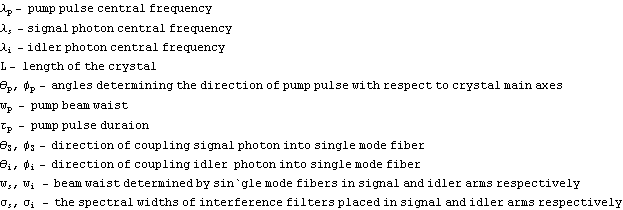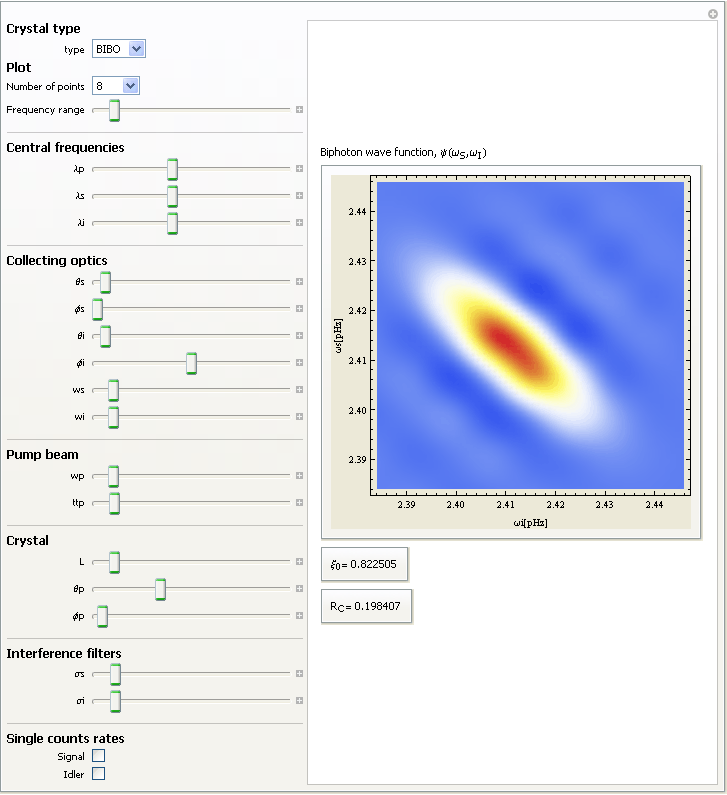# Pulsed Parametric Down Conversion

## Help

• ### Brief introduction

The nonlinear crystal of length L and cut angles Subscript[θ, p], SubscriptBox[φ, p] is pumped by pulsed gaussian beam of central wavelength Subscript[λ, p], time duration SubscriptBox[τ, p] and beam waist Subscript[w, p].The colecting optics and single mode fibers determine coupled spatial gaussian shape modes centered arround direction SubscriptBox[θ, s] SubscriptBox[φ, s] and Subscript[θ, i], Subscript[φ, i] for signal and idler arms respectively.The gaussian fiber determined modes waists are SubscriptBox[w, s] and SubscriptBox[w, i].

• ### Steereing parameters

Number of points - the numeber of rows and columns of matrix approximation of biphoton wave function
Frequency range - the range of frequencies arround signal Subscript[λ, s] and idler Subscript[λ, i] central frequencies

• ### Setup parameters## ProgramAuthor: Piotr Kolenderski
www.fizyka.umk.pl/~kolenderski

Created by Wolfram Mathematica 6.0  (25 February 2008)
Updated: 13/10/2013, 18:09